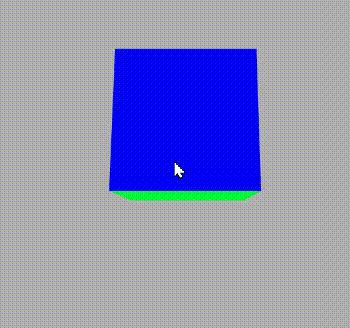GeeksforGeeks App
Open AppBrowser
Continue

# p5.js exitPointerLock() Function

The function exitPointerLock() quit the previously used Pointer Lock for example if the pointer is locked then it unlock it and vice-versa. It is used to exit the pointer lock  which is called by requestPointer() function.

Syntax:

`exitPointerLock()`

Step 1: Open the web editor of p5.js https://editor.p5js.org/

Step 2:Write the following code and see the result.

Example:

## Javascript

 `// Make a boolean variable and set``// its value false``let lock = ``false``;` `// Set the function``function` `setup() {` `    ``// Set the frame rate``    ``frameRate(5);` `    ``// Set the canvas size``    ``createCanvas(600, 400, WEBGL);``}` `// Set the draw function``function` `draw() {` `    ``// Set the background colour``    ``background(175);` `    ``// Set the camera``    ``let cX = random(-10, 10);``    ``let cY = random(-10, 10);``    ``let cZ = random(-10, 10);` `    ``camera(cX, cY,``        ``cZ + (height / 2) / tan(PI / 6),``        ``cX, 0, 0, 0, 1, 0);` `    ``ambientLight(255);` `    ``rotateX(-movedX * 0.1);``    ``rotateY(movedY * 0.1);` `    ``noStroke();``    ``normalMaterial();` `    ``box(100, 100, 100);``}` `// Function to apply exitpointer lock``function` `mouseReleased() {` `    ``// If lock is not false then``    ``// make it true``    ``if` `(!lock) {``        ``lock = ``true``;` `        ``// Request for pointer lock``        ``requestPointerLock();``    ``} ``else` `{` `        ``// Exit the pointer lock``        ``exitPointerLock();` `        ``// Again make the lock variable false``        ``lock = ``false``;``    ``}``}`

Output:In output, we can see that when the mouse is released it exit the previous pointer lock because we have set the mouseReleased() function to exit the pointer lock.

My Personal Notes arrow_drop_up
Related Tutorials